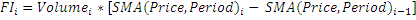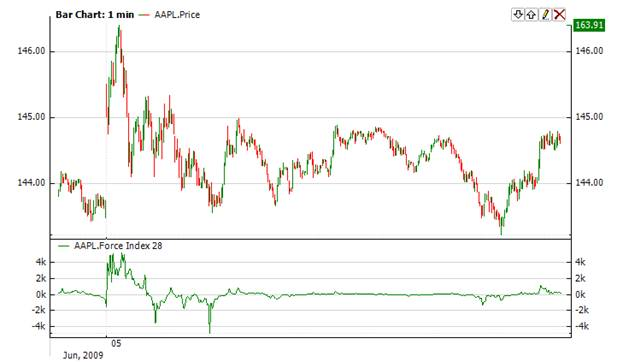﻿Force Index

# Force Index

ForceIndex measures the uptrend power at each increase, and the downtrend power at each decrease.  It connects the basic elements of market information: price trend, its strength, and volume of transactions. This index can be used as is, but it might be better to approximate it with the help of moving average.  A short moving average approximation contributes to locating the best opportunity to open and close positions.  If the approximation is made with a long moving average (for example, period 13), the Force Index shows the trends and their changes.Market Signals

It is better to buy when the forces become negative in a period of increasing indicator tendency.  In this case, the Force Index signals the continuation of the increasing trend when it rises to a new peak.  When the index becomes positive during a decreasing trend, we have a sell signal.  In this case, The Force Index signals the power of the bears and continuation of the decreasing trend as the index falls to a new trough.CalculationChart ExampleImplementation and Usage

To initialize ForceIndex use one of the constructors provided:

ForceIndex – set default values: period = 14

ForceIndex(Int32) – set value for period

ForceIndex(TimeSpan) – set time period

ForceIndex(IAverager) - sets the type of averaging

Use

FI - property to get current valueExample
C#
``` 1// Create new instance
2ForceIndex force = new ForceIndex(28);
3
4// Number of stored values
5force.HistoryCapacity = 2;
6
9
10// Get indicator value
11double IndicatorValue = force.FI;
12// Get previous value
13if (force.HistoryCount == 2)
14{
15    double IndicatorPrevValue = force;
16}
17Force Index with custom averaging:
18// Create new instance, but instead SMA we will use EMA, period = 28
19ForceIndex force = new ForceIndex(new Ema(28));
20